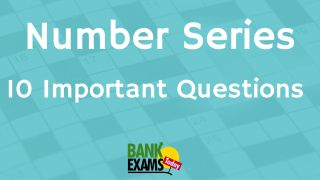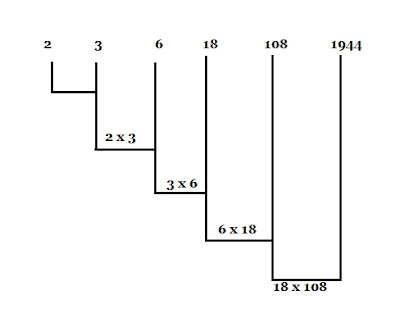New Students Offer - Use Code HELLO

# Number Series : 10 Important QuestionsOne of the number in the series is wrong, find the wrong number in the series :-

### Ques 1.

36 , 43 , 49 , 54 , 60 , 61 , 63 , 64
(a) 60
(b) 63
(c) 54
(d) 43

### Ques 2.

3 , 10 , 41 , 206 , 1236 , 8660
(a) 10
(b) 41
(c) 206
(d) 1236

### Ques 3.

12 , 20 , 38 , 42, 56 , 72
(a) 20
(b) 38
(c) 56
(d) 72

### Ques 4.

5040 , 840 , 160 , 42 , 14 , 7
(a) 7
(b) 160
(c) 14
(d) 840

### Ques 5.

81, 64 , 49 , 35 , 25 , 16
(a) 64
(b) 35
(c) 35
(d) 16

### Ques 6.

625 , 125 , 25 , 5 , 0
(a) 0
(b) 125
(c) 5
(d) 625

### Ques 7.

25 , 19 , 17 , 13 , 11 ,7
(a) 25
(b) 17
(c) 13
(d) 7

### Ques 8.

3 , 10 , 27 , 4 , 16 , 64 , 5 , 25 , 125
(a) 25
(b) 16
(c) 10
(d) 3

### Ques 9.

13, 39 , 195 , 1363 , 12285
(a) 13
(b) 1363
(c) 39
(d) 195

### Ques 10.

2 , 3, 6, 18 , 108 , 1942
(a) 6
(b) 18
(C) 1942
(d) 3

### solutions :

Ans 1. The difference between two consecutive number is decreasing  by 1 at each step.∴ 58 should be in place of 60.

Ans 2.The sequence followed in this series is :
3 x 3 + 1 = 10
10 x 4 + 1= 41
41 x 5 + 1 = 206
206 x 6 +1 = 1237; and
∴ 1237 shuold be in place of 1236.

Ans 3. The sequence followed  in this series is :
3 x 4  = 12
4 x 5  = 20
5 x 6  = 30
6 x 7  = 42
7 x 8  = 56
8 x 9  = 63
∴ 30 should be in place of 38.

Ans 4.The numbers in the series are divided by numbers in reverse  order starting from 6 to get the next number .∴ 168 should be in place of 32.

Ans 5. The sequence is the  square of the number in decreasing order  starting from 9, i.e
92  = 9 x 9 = 81
82  = 8 x 8 = 64
72  = 7 x 7 = 49
62  = 6 x 6 = 64
52  = 5 x 5 = 25
42  =  4 x 4 = 16
∴ 36 should be in place of 35

Ans 6 . The number in the series are divided by 5 to get the next number .∴ 1 should be in place of 0 digit.

Ans 7. Other numbers are prime numbers.

Ans 8. The sequence of the series is formed by a  number ,its square , its cube, then the next  number is natural order , i.e.,
31  = 3
32  = 3 x 3 = 9
33  = 3  x 3 x 3 = 27
next number :
41 = 4
4= 4 x 4  = 16
43 =  4 x 4 x 4 = 64
next number :
51 = 5
52  = 5 x 5 = 25
53  = 5 x 5 x 5 = 125
∴   9 should be in place of 10 .
Ans 9. In this series the numbers are multiplied by odd numbers in natural order numbers in natural order starting from 3, i .e.∴ 1365 should be in place of 1363

Ans 10. The numbers in the series are the product of two numbers preceding it , i.e.∴ 1944 should be in place 0f 1942.